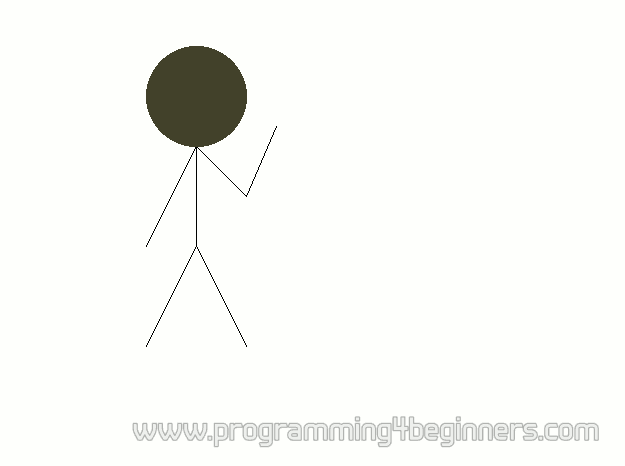## Computing a Picture

### Drawing Lines

As usual, the column number will be represented by the coordinate x, and the row number will be represented by the coordinate y. The only unusual thing is that the coordinate y grows downwards. The two coordinates x and y can be represented by a coordinate pair written in parenthesis like this: (x, y).

The next question that naturally comes to mind is how we should draw a line between two chosen points? The points in question can be specified by their coordinate pairs. The desired line would then go from a point at coordinates (x1, y1) to a point at coordinates (x2, y2). To calculate the intermediate points, we would need to use the equation of a line and a lot of mathematics; fortunately, the people who wrote the Allegro library have already done this work for us. To draw a line, we just need to call a function that has the following form:

```line(surface, x1, y1, x2, y2, color)
```

For example, to draw a line from a point (pixel) having coordinates (30, 40) to a point (pixel) having coordinates (270, 420) and in the red color, we would use the statement:

```line(surface, 30, 40, 270, 420, makecol(255,0,0) );
```For demonstration purposes, here we give a program that draws a few lines and a circle:

```#include <allegro.h>

int main()
{
if (allegro_init()!=0) return 1;
set_color_depth(32);
if (set_gfx_mode(GFX_AUTODETECT_WINDOWED, 800, 600, 0, 0)!=0)
return 2;
BITMAP* surface = create_bitmap(SCREEN_W, SCREEN_H);
install_keyboard();
clear_to_color(surface, makecol(255,255,255));
line(surface,200,150,200,250,makecol(0,0,0));
line(surface,200,250,150,350,makecol(0,0,0));
line(surface,200,250,250,350,makecol(0,0,0));
line(surface,200,150,150,250,makecol(0,0,0));
line(surface,200,150,250,200,makecol(0,0,0));
line(surface,250,200,280,130,makecol(0,0,0));
circlefill(surface,200,100,50,makecol(64,64,64));
blit(surface, screen, 0,0, 0,0, SCREEN_W,SCREEN_H);
while (!keypressed())
rest(20);
return 0;
}
END_OF_MAIN()
```

This program will produce a simplified drawing of a human. He is waving, so why do you not wave a hand to him, too.

In the previous program, the statement

```circlefill(surface,200,100,50,makecol(64,64,64));
```

draws a circle having a center on coordinates (200, 100) and a radius of 50 pixels.